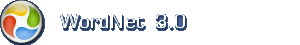# Engelsk ordbog

Tip: Spørgsmålstegn (?) kan anvendes som jokertegn (wild card). Spørgsmålstegnet erstatter præcis et tegn.

## Engelsk navneord: mathematical function

1. mathematical function (mathematics) a mathematical relation such that each element of a given set (the domain of the function) is associated with an element of another set (the range of the function)

Termer med samme betydning (synonymer)function, map, mapping, single-valued function

Mindre specifikke termermathematical relation

Mere specifikke termercircular function, expansion, exponential, exponential function, inverse function, isometry, Kronecker delta, metric, metric function, multinomial, operator, polynomial, threshold function, transformation, trigonometric function

Overordnet emneområdemath, mathematics, maths

Baseret på WordNet 3.0 copyright © Princeton University.
Teknik og design: Orcapia v/Per Bang. Dansk bearbejdning: .
2019 onlineordbog.dk